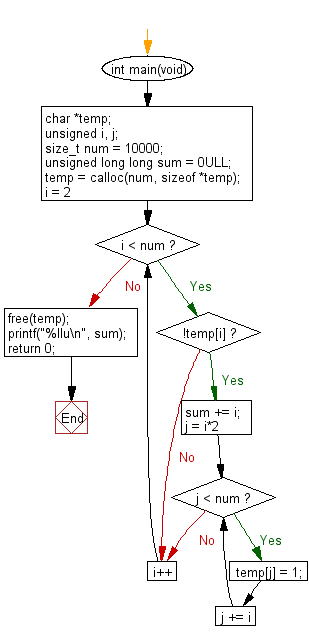﻿ C Program: Find the sum of all the primes below ten thousand - w3resource# C Exercises: Find the sum of all the primes below ten thousand

## C Programming Practice: Exercise-26 with Solution

A prime number is a natural number greater than 1 that cannot be formed by multiplying two smaller natural numbers. A natural number greater than 1 that is not prime is called a composite number. For example, 5 is prime because the only ways of writing it as a product, 1 × 5 or 5 × 1, involve 5 itself. However, 6 is composite because it is the product of two numbers (2 × 3) that are both smaller than 6.
The sum of the primes below 10 is 2 + 3 + 5 + 7 = 17.
Write a C programming to find the sum of all the primes below ten thousand.

C Code:

``````#include <stdio.h>
#include <stdlib.h>
int main(void)
{
char *temp;
unsigned i, j;
size_t num = 10000;
unsigned long long sum = 0ULL;

temp = calloc(num, sizeof *temp);
for (i = 2; i < num; i++) {
if (!temp[i]) {
sum += i;
for (j = i*2; j < num; j += i) {
temp[j] = 1;
}
}
}
free(temp);
printf("%llu\n", sum);
return 0;
}
``````

Sample Output:

```5736396
```

Flowchart:## C Programming Code Editor:

What is the difficulty level of this exercise?

Test your Programming skills with w3resource's quiz.

﻿

## C Programming: Tips of the Day

Maximum value of int:

In C:

```#include <limits.h>
then use
int imin = INT_MIN; // minimum value
int imax = INT_MAX;```

or

```#include <float.h>

float fmin = FLT_MIN;  // minimum positive value
double dmin = DBL_MIN; // minimum positive value

float fmax = FLT_MAX;
double dmax = DBL_MAX;```

Ref : https://bit.ly/3fi8yk9